# JP5385740B2 - Imaging apparatus and image data correction method - Google Patents

## Info

Publication number
JP5385740B2
JP5385740B2 JP2009221443A JP2009221443A JP5385740B2 JP 5385740 B2 JP5385740 B2 JP 5385740B2 JP 2009221443 A JP2009221443 A JP 2009221443A JP 2009221443 A JP2009221443 A JP 2009221443A JP 5385740 B2 JP5385740 B2 JP 5385740B2
Authority
JP
Japan
Prior art keywords
means
optical member
correction information
correction
optical
Prior art date
Legal status (The legal status is an assumption and is not a legal conclusion. Google has not performed a legal analysis and makes no representation as to the accuracy of the status listed.)
Active
Application number
JP2009221443A
Other languages
Japanese (ja)
Other versions
JP2011071766A (en
JP2011071766A5 (en
Inventor

Original Assignee
キヤノン株式会社
Priority date (The priority date is an assumption and is not a legal conclusion. Google has not performed a legal analysis and makes no representation as to the accuracy of the date listed.)
Filing date
Publication date
Application filed by キヤノン株式会社 filed Critical キヤノン株式会社
Priority to JP2009221443A priority Critical patent/JP5385740B2/en
Publication of JP2011071766A publication Critical patent/JP2011071766A/en
Publication of JP2011071766A5 publication Critical patent/JP2011071766A5/ja
Application granted granted Critical
Publication of JP5385740B2 publication Critical patent/JP5385740B2/en
Application status is Active legal-status Critical
Anticipated expiration legal-status Critical

## Images

•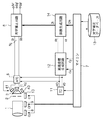•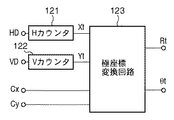•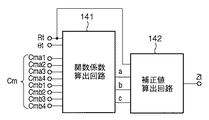•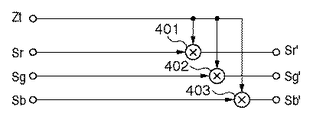•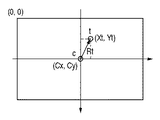•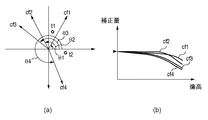•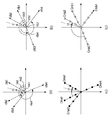•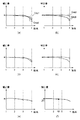•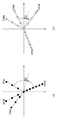•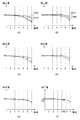•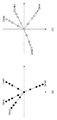•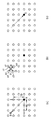•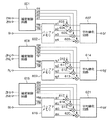•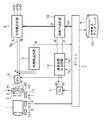•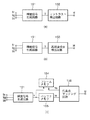•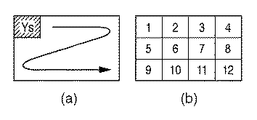•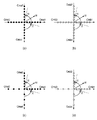## Classifications

• HELECTRICITY
• H04ELECTRIC COMMUNICATION TECHNIQUE
• H04NPICTORIAL COMMUNICATION, e.g. TELEVISION
• H04N5/00Details of television systems
• H04N5/30Transforming light or analogous information into electric information
• H04N5/335Transforming light or analogous information into electric information using solid-state image sensors [SSIS]
• H04N5/357Noise processing, e.g. detecting, correcting, reducing or removing noise
• H04N5/3572Noise processing, e.g. detecting, correcting, reducing or removing noise the noise originating only from the lens unit, e.g. flare, shading, vignetting or "cos4"

## Description

The present invention relates to an imaging apparatus and a method for correcting image data, and more particularly to a technique for correcting image quality degradation due to optical factors based on optical information.

In recent digital video cameras and digital cameras, the number of pixels has increased, and it has become possible to obtain higher-resolution and higher-quality images. On the other hand, due to factors such as image degradation due to pixel miniaturization and difficulty in designing a small lens, the image quality that the optical system causes in principle, such as a reduction in the amount of light around the image circle, chromatic aberration of magnification, and distortion, etc. Deterioration is becoming apparent.

The above-described deterioration in image quality due to optical factors is theoretically considered to have point-symmetric phenomenon characteristics that radiate from the center of the optical axis. However, since the actual image is affected by the characteristics of the lens composed of multiple lens groups, the shape of the optical member such as the aperture mechanism, and the physical structure of the sensor, the image quality is not necessarily point-symmetric. It does not always result in deterioration.

Conventionally, a technique for correcting image quality deterioration having such asymmetry phenomenon characteristics by using optical system parameter information (hereinafter referred to as “optical parameters”) such as focal length, aperture, and focus position. Has been proposed (see, for example, Patent Documents 1 to 4).

In particular, as in a video camera, when it is assumed that the focal length, the aperture, and the focus position constantly change during shooting, the image quality degradation due to optical factors sequentially changes due to changes in optical parameters. In addition, resources such as the memory capacity necessary for holding the correction characteristic data for correcting the above-described astigmatism image quality degradation and the time for processing the correction characteristic data are considered when taking a picture with a camera or a video camera. It is necessary to consider that it is finite and few.

In order to deal with such a situation, when generating the correction characteristic data, it is necessary to appropriately control according to the state of the optical characteristic and the photographing situation while taking into consideration the astigmatism of the image quality degradation.

Japanese Patent No. 3824237 Japanese Patent No. 3912404 JP-A-2005-341033 JP 2007-134903 A

In Patent Document 1, a mixing ratio of optical correction values is determined according to an optical parameter, and a shading correction is performed according to a pixel distance obtained by correcting the distance from the screen center of a target pixel in the vertical and horizontal directions with a distance gain function. Is going. However, the basic correction characteristics are simply changed by the distance gain function. Therefore, it is necessary to have a complex distance gain function for optical characteristics with complicated astigmatism, and it is not possible to cope with shape changes that do not have similarities. Can't get. In addition, approximating correction characteristics for changes in optical parameters and image height position with a simple model does not actually realize proper correction processing.

In Patent Document 2, the relative position between the center of the optical axis and the center of the image sensor due to image stabilization is detected, and the phase of shading correction information that is discretely held radially from the center of the optical axis is switched. However, in this example, since the discretely arranged correction information is used as it is, the density of the correction information can only be increased for optical characteristics having complicated asymmetry.

In Patent Document 3, in order to deal with the shading asymmetry caused by the sensor structure, the correction information held in the x-axis and y-axis directions is given asymmetry, and the correction coefficient at the target pixel position is calculated. . However, in this example, since the asymmetric nature of the entire screen is expressed by synthesizing the line-symmetric phenomenon characteristics that can be expressed by a specific function, the optical characteristics change in the angular direction with respect to the optical axis center. Can not respond.

In Patent Document 4, in order to deal with asymmetry of shading caused by distortion of the optical system, correction information is converted with an actually measured shading amount, and a correction coefficient at a target pixel position is calculated. However, in this example, it is necessary to actually measure the asymmetry characteristics and hold the information, but if based on actual measurement, there is essentially no significance of having the correction information itself. Further, it is practically impossible to perform the same process under actual shooting conditions in which optical parameters change sequentially.

The present invention has been made in view of the above problems, and an object of the present invention is to make it possible to quickly correct image quality deterioration due to optical factors having complicated asymmetry without increasing the apparatus configuration. And

In order to achieve the above object, an imaging apparatus according to the present invention includes a control unit that controls an optical member using a parameter, an imaging unit that generates image data by converting light imaged through the optical member , and , due to the characteristics of the optical member, a point-asymmetrical about the optical axis of the optical member, a correction information for correcting the image degradation of the image data, a polar coordinate system centered on the optical axis of the optical member a storage means for the correction information corresponding to a plurality of image heights of the plurality of polarization angles, and stores the plurality of values parameter can take to control the optical member in,
When said control means controls said optical member by using the parameter, wherein among the stored correction information storage means, the correction corresponding to the value of the near Ifuku number to the value of the parameter the control means is used reading means for reading the information, the pixel position of the image data generated by the imaging unit, a conversion unit that converts the polar coordinates around the optical axis of the optical member, and the values of the parameters the control means is used The correction information corresponding to the polar coordinates converted by the conversion means is generated by interpolating the correction information read by the reading means, and the correction information generated by the generation means is used to generate the correction information. have a correction means for correcting the image data of the polar coordinates of the pixel position, the storage means has a large degree of change in the image quality deterioration at the center and the circumferential direction of the optical axis of the optical member As circumference, stores correction information corresponding to the number of declination.

According to the present invention, it is possible to quickly correct image quality degradation due to an optical factor having complicated asymmetry without increasing the apparatus configuration.

1 is a block diagram showing a schematic configuration of an imaging apparatus according to a first embodiment. The block diagram which shows the internal structure of the pixel coordinate generation circuit concerning 1st Embodiment. The block diagram which shows the internal structure of the coefficient generation circuit concerning 1st Embodiment. 1 is a block diagram showing a configuration example of an optical correction circuit according to a first embodiment. The figure which shows the example which expressed the pixel position in an imaging device by the polar coordinate. The figure which shows an example of the corrected amount with respect to the optical characteristic which has astigmatism with respect to image height. The figure for demonstrating an example of the optical correction characteristic information which has astigmatism hold | maintained at the optical correction database concerning 1st Embodiment. The figure for demonstrating the calculation method of the correction coefficient concerning 1st Embodiment. FIG. 10 is a diagram for explaining another example of optical correction characteristic information according to the first modification. The figure for demonstrating the calculation method of the correction coefficient concerning the modification 1. FIG. FIG. 10 is a diagram for explaining another example of optical correction characteristic information according to Modification 2. The schematic diagram for demonstrating the process of the magnification chromatic aberration correction circuit or distortion aberration correction circuit concerning 2nd Embodiment. The block diagram which shows the structural example of the optical correction circuit concerning 2nd Embodiment. The block diagram which shows schematic structure of the imaging device concerning 3rd Embodiment. FIG. 9 is a block diagram illustrating a configuration example of a feature detection circuit according to a third embodiment. The figure for demonstrating the acquisition method of the feature evaluation value by matching. The figure for demonstrating an example of the optical correction characteristic information concerning 2nd implementation.

The best mode for carrying out the present invention will be described below in detail with reference to the accompanying drawings.

<First Embodiment>
FIG. 1 is a block diagram illustrating a schematic configuration of the imaging apparatus according to the first embodiment. In FIG. 1, 1 is a lens unit including a zoom lens and a focus lens, and 2 is a stop. When the imaging device is a video camera, the optical system including the lens unit 1 and the diaphragm 2 has various functions such as zooming operation, movement of the subject, change of the shooting target, environmental change of ambient brightness, and shooting effect intended by the photographer. Is controlled according to various factors. That is, the optical parameters represented by the focal length, the focus position, and the aperture value are always controlled and fluctuated.

The light that has passed through the optical unit including the lens unit 1 and the diaphragm 2 is color-separated into red (R), green (G), and blue (B) by the color separation prism 3 and is optically applied to the image sensors 41 to 43, respectively. The image is formed as a simple image and sequentially converted into an image signal. Image signals output from the image sensors 41 to 43 are converted into digital image signals Sr, Sg, and Sb by an analog front end (AFE) 5.

The digital image signals Sr, Sg, Sb are input to the optical correction circuit 6, and correction values Zt input from a coefficient generation circuit 14 described later are sequentially applied to each pixel. Thereby, the optical phenomenon caused by the lens unit 1 and the diaphragm 2 is corrected, and digital image signals Sr ′, Sg ′, Sb ′ are output.

Reference numeral 7 denotes a microcomputer that controls the operation of the entire imaging apparatus.

Reference numeral 8 denotes an actuator for driving the zoom lens of the lens unit 1, and drives the zoom lens based on the drive pulse CL output from the microcomputer 7. An actuator 9 drives the focus lens of the lens unit 1 and drives the focus lens based on the drive pulse CF output from the microcomputer 7. An actuator 10 for driving the diaphragm 2 drives the diaphragm 2 based on a drive pulse Cf output from the microcomputer 7.

The timing generator (TG) 11 generates a horizontal synchronization signal HD and a vertical synchronization signal VD in accordance with the drive specifications of the image sensors 41 to 43 and supplies them to the AFE 5 and a pixel coordinate generation circuit 12 described later. The AFE 5 generates drive signals for the image sensors 41 to 43 based on the horizontal synchronization signal HD and the vertical synchronization signal VD and inputs the drive signals to the image sensors 41 to 43.

The pixel coordinate generation circuit 12 generates polar coordinates of the current pixel based on the optical axis position information Cx, Cy supplied from the microcomputer 7 and the horizontal synchronization signal HD and the vertical synchronization signal VD supplied from the TG 11. This is supplied to the coefficient generation circuit 14. Image quality degradation caused by the optical system includes optical aberrations such as chromatic aberration of magnification and distortion, and a decrease in the amount of peripheral light. These are known to vary depending on the image height. Therefore, the pixel positions in the image sensors 41 to 43 obtained based on the horizontal synchronization signal HD and the vertical synchronization signal VD are expressed by the pixel coordinate generation circuit 12 by the radius Rt from the optical axis and the deflection angle θt from the start line. To polar coordinates. Details of generation of polar coordinates will be described later.

Reference numeral 14 denotes a coefficient generation circuit which performs optical correction based on polar coordinates obtained from the pixel coordinate generation circuit 12 and optical correction data Cm (correction information) stored in the optical correction database 13 input via the microcomputer 7. The circuit 6 generates a correction value Zt for correcting an optical phenomenon. The optical correction database 13 is a storage device such as a flash memory.

Next, processing for generating the correction value Zt in the imaging apparatus having the above configuration will be described in detail.

Generation of Polar Coordinates FIG. 2 is a block diagram showing a detailed configuration of the pixel coordinate generation circuit 12. As shown in FIG. 2, the H counter 121 reset at the falling edge of the horizontal synchronizing signal HD and the V counter 122 reset at the falling edge of the vertical synchronizing signal VD respectively set the horizontal counter value Xt and the vertical counter value Yt. Output. As shown in FIG. 5, the horizontal counter value Xt and the vertical counter value Yt obtained here are Xt in the horizontal direction and the vertical direction in the vertical direction with the coordinates of the pixel at the upper left corner of the image sensor 41 to 43 as (0, 0). The coordinates (Xt, Yt) of the pixel of Yt are shown.

The optical axis position information Cx, Cy supplied from the microcomputer 7 indicates at which position (coordinates) the optical axis c of the optical system composed of the lens unit 1 and the diaphragm 2 is located in the imaging elements 41 to 43.

The polar coordinate conversion circuit 123 converts the horizontal counter value Xt and the vertical counter value Yt of the pixel t into a polar coordinate system with the optical axis C as the origin, based on the optical axis position information Cx and Cy. Polar coordinates are

Therefore, the polar coordinate conversion circuit 123 calculates the above formula and outputs polar coordinate values Rt and θt. Note that the square root operation is expressed by a known method such as a bisection method or a square root method with a finite word length accuracy, and the atan operation is a high-order function approximation or a divided low-order that divides the XY ratio into predetermined ranges. It can also be realized in hardware by function approximation.
Selection of correction amount plot data set As described above, the optical characteristics of the lens unit 1 and the aperture stop 2 including a plurality of lenses may be asymmetric with respect to the shape of the optical member, the physical structure of the sensor, and the like. Many. 6A shows the radial directions cf1 to cf4 of the declination angles θ1 to θ4 about the optical axis c, and FIG. 6B shows the radial directions cf1 to cf4 shown in FIG. 6A. 2 shows an example of a correction amount for an appropriate optical characteristic at an image height. In the example shown in FIG. 6, it is assumed that the optical characteristics have asymmetry that varies depending on the angle, and the correction amounts in the radial directions Cf1 to Cf4 do not match each other as shown in FIG. 6B.

In the first embodiment, in order to obtain a correction amount for correcting image quality degradation due to such astigmatic optical characteristics, optical correction characteristic information and area information for optical parameters are previously stored in an optical correction database. 13 is held. The optical correction characteristic information held by the optical correction database 13 is, for example, plot data that directly indicates correction amounts (plot data) at a plurality of preset image heights (radial radius) in a radial direction at a plurality of declinations. Is a set. Instead of the plot data set, a coefficient term of a function approximating the characteristic of the correction amount in each radial direction may be held. The area information held in the optical correction database 13 is polar coordinates (declination and image height) around the optical axis at the position corresponding to each of the held correction amounts.

FIG. 7 is a diagram illustrating an example of a process for obtaining optical correction data to be input to the coefficient generation circuit 14 from the optical correction characteristic information held in the optical correction database 13 in the first embodiment.

FIG. 7A shows four radial directions cfa1 to cfa4 and their declination angles θa1 to θa4 that hold the optical correction characteristic information corresponding to the optical parameter Oa. The seventh (b) represents the four radial directions cfb1 to cfb4 holding the optical correction characteristic information and the deflection angles θb1 to θb4 corresponding to the optical parameter Ob. Thus, in the first embodiment, optical correction characteristic information is held for each of a plurality of different optical parameters.

FIGS. 7C and 7D show positions corresponding to the respective correction amounts of the optical correction characteristic information corresponding to the optical parameter Oa and the optical parameter Ob. As can be seen from FIGS. 7A and 7C, the optical correction characteristic information corresponding to the optical parameter Oa has a total of 13 pieces of correction (correction in the optical axis) for each of the four radial directions cfa1 to cfa4. The amount is common). A set of correction amounts (plot data) for each of the radial directions cfa1 to cfa4 and region information (declination angles θa1 to θa4 and image height) corresponding to each correction amount is hereinafter referred to as correction amount plot data sets Cma1 to Cma4. Call it. The correction amount plot data sets Cma1 to Cma4 are collectively referred to as a correction amount plot data set Cma. Further, four image heights are set to image heights 1 to 4 from the optical axis toward the periphery, and correction amounts (plot data) included in the correction amount plot data set Cma1 are referred to as correction amount plot data Cma11 to Cma14. The correction amount plot data included in the correction amount plot data sets Cma <b> 2 to Cma <b> 4 are similarly expressed.

Similarly, as can be seen from FIGS. 7B and 7D, the optical correction characteristic information corresponding to the optical parameter Ob is also set to four image heights for each of the four radial directions cfb1 to cfb4, for a total of 13 (light The correction amount on the axis is common). A set of correction amounts (plot data) for each of the radial directions cfb1 to cfb4 and region information (declination angles θb1 to θb4 and image height) corresponding to each correction amount is hereinafter referred to as correction amount plot data sets Cmb1 to Cmb4. Call it. The correction amount plot data sets Cmb1 to Cmb4 are collectively referred to as a correction amount plot data set Cmb. Further, the correction amounts (plot data) included in the correction amount plot data set Cmb1 are referred to as correction amount plot data Cmb11 to Cmb14 with the four image heights from the optical axis toward the periphery as image heights 1 to 4. The same applies to the correction amount plot data included in the correction amount plot data sets Cmb2 to Cmb4.

In FIG. 7, the reason why the correction amount corresponding to four image heights is not held in each of the radial directions of the four declinations is because the memory capacity of the optical correction database 13 is finite, and the correction amount is only discrete. I can't. In the first embodiment, the optical correction characteristic information for three radial directions is obtained for the range of declination where the optical characteristic of astigmatism is drastically changed, and for one radial direction for the range of gentle declination. Allocated in advance. Therefore, in the optical parameter region, image height position, and declination where there is no correction amount, the correction amount is acquired by interpolating from neighboring information. Note that the direction and number of the optical correction characteristic information of the present invention held in the optical correction database 13 is not limited to this, and depends on the memory capacity allowed by the imaging apparatus and the tendency of the characteristic change amount of the optical system. Thus, the amount of information can be determined in advance.

On the other hand, the control unit time based on the optical parameter (image capturing period of one image) is one cycle (one frame period) of the vertical synchronization signal VD indicating the update of the image signal, so the processing time that the microcomputer 7 gives to the optical correction process is Of course it is finite. Therefore, the microcomputer 7 acquires a plurality of sets of finite number of plot data and area information that can be processed during one frame period from those corresponding to the optical parameter close to the current optical parameter Ot.

Therefore, the microcomputer 7 first selects two optical parameters in the vicinity of the current optical parameter Ot from among the plurality of optical parameters of the optical correction characteristic information held in the optical correction database 13. Here, the optical parameters Oa and Ob described above are used. Then, the microcomputer 7 outputs correction amount plot data sets Cma and Cmb corresponding to the selected optical parameters Oa and Ob to the coefficient generation circuit 14 as optical correction data Cm. The optical correction data Cm is output when the microcomputer 7 outputs drive pulses CL, CF, and Cf to the optical unit 1 and the diaphragm 2.

Generation of Correction Coefficient FIG. 3 is a block diagram showing an example of the configuration of the coefficient generation circuit 14. The polar coordinate values Rt and θt of the current processing target pixel output from the pixel coordinate generation circuit 12 and the optical correction data Cm output from the microcomputer 7 are input to the function coefficient calculation circuit 141. Here, a case where the pixel t1 (radius = Rt1, declination = θt1) in FIG. 7 is processed will be described as an example.

The function coefficient calculation circuit 141 corrects two corrections corresponding to the deflection angles θa1 and θa2 sandwiching the deflection angle θt1 of the pixel t1 among the input correction amount plot data sets Cma1 to Cma4 as shown in FIG. 7C. The quantity plot data sets Cma1, Cma2 are selected. FIG. 8A shows correction amount plot data for four image heights (1 to 4) of the selected correction amount plot data sets Cma1 and Cma2. The function coefficient calculation circuit 141 calculates two correction amount plot data given for each image height as the distance between the deflection angle θt1 of the pixel t1 and the deflection angles θa1 and θa2 of the correction amount plot data sets Cma1 and Cma2. Is weighted and interpolated. As a result, a weighted average value of the four correction amount plot data as shown in FIG. 8C is calculated.

Similarly, as shown in FIG. 7D, the function coefficient calculation circuit 141 receives two correction angle plot data sets Cmb1 to Cmb4 corresponding to two deflection angles θb2 and θb3 sandwiching the pixel t1 deflection angle θt1. The correction amount plot data sets Cmb2 and Cmb3 are selected. FIG. 8B shows correction amount plot data for four image heights (1 to 4) of the selected correction amount plot data sets Cmb2 and Cmb3. The function coefficient calculation circuit 141 weights the two correction amount plot data given for each image height by the distance between the deflection angle θt1 of the pixel t1 and the deflection angles θb2 and θb3 of the correction amount plot data set Cma2. And interpolate. As a result, a weighted average value of the four correction amount plot data as shown in FIG. 8D is calculated.

Next, the function coefficient calculation circuit 141 further averages the weighted average values of the correction amount plot data shown in FIGS. 8C and 8D for each image height, and performs correction as shown in FIG. Obtain the average value of the quantity plot data.

Finally, as shown in FIG. 8F, the function coefficient calculation circuit 141 obtains an image height section to which the radius Rt1 of the pixel t1 belongs (in this example, between the image heights 2 and 3), and obtains the obtained image. The coefficients a, b, and c of the approximate function corresponding to the high section are output.

The correction value calculation circuit 142 calculates the correction amount Zt1 from the coefficients a, b, and c and the image height Rt1 of the pixel t1 based on the following equation.
Zt1 = aRt1 2 + bRt1 + c

In the first embodiment, the image height section is approximated by a quadratic function as shown in the above formula. However, this is a linear function (polygonal line approximation), or a higher order higher than the third order. It is also possible to approximate with a function.

The correction amount Zt1 for correcting the optical characteristic of the current processing target pixel t1 thus obtained is output from the correction value calculation circuit 142 of the coefficient generation circuit 14 to the optical correction circuit 6. The correction amount Zt is sequentially obtained by the above-described procedure for each pixel t and is output to the optical correction circuit 6.

Correction of Optical Phenomenon FIG. 4 is a diagram showing a configuration example of the optical correction circuit 6. Here, description will be made assuming that the peripheral light amount drop is corrected as an optical characteristic. In the case of the peripheral light amount drop, the correction amount Zt output from the coefficient generation circuit 14 is a gain value with the light amount drop amount at the position of the processing target pixel as an inverse number.

The correction amount Zt input to the optical correction circuit 6 is multiplied by the digital image signals Sr, Sg, Sb output from the AFE 5 by multipliers 401, 402, 403, respectively, and the digital image signal Sr ′ subjected to optical correction processing. , Sg ′, Sb ′ are output.

As described above, according to the first embodiment, it is possible to quickly correct image quality degradation due to astigmatic optical characteristics without increasing the necessary memory capacity.

As described above, the optical correction database 13 may hold a coefficient term of a function approximating the correction amount characteristic instead of plot data directly indicating the correction amount of the optical characteristic. In this case, the “correction amount plot data” described above may be replaced with the order of the approximate function.

<Modification 1>
In the first embodiment described above, the correction amount plot data in each radial direction included in the correction amount plot data sets Cma and Cmb is uniformly four as shown in FIG. On the other hand, as shown in FIG. 9, in the direction in which the change in astigmatism is gentle (Cma4 and Cmb3), the interpolation calculation accuracy can be improved by assigning more correction amount plot data. As an example, a case will be described in which the correction coefficient of the pixel t2 (radial radius = Rt2, declination = θt2) in FIG. 9 is obtained. In the first modification, it is assumed that the correction amount plot data sets Cma and Cmb are selected based on the optical parameters as in the first embodiment.

As can be seen from FIG. 9A, the function coefficient calculation circuit 141 includes two correction amount plot data sets Cma1 sandwiching the pixel t2 based on the deflection angle θt2 of the pixel t2, among the correction amount plot data sets Cma1 to Cma4. , Cma4 is selected. In this case, as shown in FIG. 10A, four and seven correction amount plot data are included, respectively. When there are two correction amount plot data at the same image height among the correction amount plot data given for each image height, the deflection angle θt2 of the pixel t2 and the correction amount plot data sets Cma1, Cma4. Are interpolated by weighting them with the distances of the deviation angles θa1 and θa4. For image heights having only one correction amount plot data (image heights 2, 4, and 6), first, correction amount plot data Cma12, Cma14, and Cma16 of the correction amount plot data set Cma1 are obtained at the front and rear image heights. The correction amount plot data is averaged. Then, the obtained value and the correction amount plot data Cma42, Cma44, and Cma46 of the correction amount plot data set Cma4 are subjected to weighted interpolation processing. As a result, the weighted average value of the eight correction amount plot data as shown in FIG. 10C is calculated.

On the other hand, the function coefficient calculation circuit 141 selects two correction amount plot data sets Cmb1 and Cmb4 sandwiching the pixel t2 based on the deflection angle θt2 of the pixel t2 among the correction amount plot data sets Cmb1 to Cmb4. FIG. 9B shows correction amount plot data for four image heights of the selected correction amount plot data sets Cmb1 and Cmb4. Then, the function coefficient calculation circuit 141 weights the two correction amount plot data at each image height by the distance between the deflection angle θt2 of the pixel t2 and the deflection angles θb1 and θb4 of the correction amount plot data sets Cmb1 and Cma4. To interpolate. As a result, the weighted average value of the four correction amount plot data as shown in FIG.

Next, the function coefficient calculation circuit 141 further averages the weighted average values of the correction amount plot data shown in FIGS. 10C and 10D for each image height, and performs correction as shown in FIG. Obtain the average value of the quantity plot data. In this example, the weighted average values of the correction amount plot data sets Cmb1 and Cmb4 do not correspond to the image heights 2, 4, and 6. For this reason, first, the weighted average values of the image heights 2, 4, and 6 are obtained from the weighted average values at the preceding and following image heights, and then averaged with the weighted average values of the correction amount plot data sets Cma1 and Cma4.

Finally, as shown in FIG. 10F, the function coefficient calculation circuit 141 obtains an image height section to which the moving radius Rt2 of the pixel t2 belongs (in this example, between the image heights 5 and 6) and obtains the obtained image. The coefficients a, b, and c of the approximate function corresponding to the high section are output.

The correction value calculation circuit 142 calculates the correction amount Zt2 from the coefficients a, b, and c and the moving radius Rt2 of the pixel t2 based on the following equation.
Zt2 = aRt2 2 + bRt2 + c

In the first modification, the image height section is approximated by a quadratic function as shown in the above formula, but this is a linear function (polygonal line approximation) or a higher-order function of the third or higher order. It is also possible to approximate.

The correction amount Zt2 for correcting the optical characteristic of the current processing target pixel t2 thus obtained is output from the correction value calculation circuit 142 of the coefficient generation circuit 14 to the optical correction circuit 6.

<Modification 2>
In the first embodiment and the first modification described above, the image heights of the correction amount plot data included in the correction amount plot data sets Cma and Cmb are equally spaced. However, as shown in FIGS. 11A and 11B, interpolation is performed by assigning a large amount of correction plot data to an image height region where the change in astigmatism becomes significant (in this drawing, a region having a high image height). Calculation accuracy can be increased.

The method for obtaining the correction coefficient using the correction plot data sets Cma and Cmb shown in FIGS. 11A and 11B is the same as the method described with reference to FIG. 8 in the first embodiment. Therefore, the description is omitted here.

<Second Embodiment>
In the first embodiment, the case where the peripheral light amount drop is corrected as the optical characteristic has been described. In the second embodiment, a case will be described in which lateral chromatic aberration and distortion are corrected. Since the overall apparatus configuration is the same as that shown in FIG. 1, the description thereof is omitted here.

The lateral chromatic aberration correction and the distortion aberration correction are processes for rearranging the image data obtained by a certain pixel to the pixel position (coordinate) that should be originally obtained when there is no lateral chromatic aberration or distortion. It is not a correction process. Accordingly, in the second embodiment, the movement direction and movement distance of the pixel (coordinates) are held as the correction amount plot data.

The correction coefficient Zt is a shift amount obtained by separating the shift amount of the pixel position as a shift component in the horizontal and vertical directions. Hereinafter, the coefficient of the shift component in the horizontal direction for red (R) is ZtHr, and the vertical direction. The coefficient of the deviation component is ZtVr. In addition, the horizontal shift component coefficient for green (G) is ZtHg, the vertical shift component coefficient is ZtVg, the horizontal shift component coefficient for blue (B) is ZtHb, and the vertical shift component coefficient is ZtHg. Let the coefficient be ZtVb. Therefore, in the second embodiment, as the optical correction characteristic information, there are correction amount plot data for the respective colors of R, G, and B in the horizontal direction and the vertical direction. Then, the correction coefficients ZtHr, ZtVr, ZtHg, ZtVg, ZtHb and ZtVb can be obtained by obtaining the correction coefficients independently for each color in each direction as described above.

FIG. 12 is a diagram showing the concept of correcting this pixel position shift. In FIG. 12A, a black circle indicates a pixel position S to be processed, and a gray circle indicates that an image signal that should originally be obtained at the pixel position S is misaligned due to the influence of lateral chromatic aberration or distortion. This is a virtual pixel S ′ indicating the position that has been set.

In order to correct lateral chromatic aberration and distortion, a virtual pixel S ′ whose phase is shifted by Hp in the horizontal direction and Vp in the vertical direction may be obtained and rearranged at the position of the pixel of interest S.

As shown in FIG. 12B, the virtual pixel S ′ includes the pixels s1, s2, s3, s4 and the virtual pixel S ′ from the pixels s1, s2, s3, s4 from which the image signals that exist in the vicinity are actually obtained. And inter-pixel distances c1, c2, c3, and c4. The generated virtual pixel S ′ is replaced with the pixel position S as shown in FIG. 12C, whereby the lateral chromatic aberration and distortion are corrected.

FIG. 13 is a block diagram showing a configuration when the optical correction circuit 6 is a magnification chromatic aberration correction circuit or a distortion aberration correction circuit for realizing the processing shown in FIG.

First, R component correction will be described. The correction coefficients ZtHr and ZtVr input to the optical correction circuit 6 are input to the interpolation control circuit 601, and of these, the integer part is output as Hrp and Vrp. Further, the interpolation control circuit 601 converts the decimal part into the conversion coefficients cr1, cr2, cr3, cr4 by weighting the inter-pixel distances between the reference pixels sr1, sr2, sr3, sr4 and the virtual pixel Sr ′ and outputs them.

The buffer memory 602 holds the digital image signal Sr sequentially over a plurality of pixels in the horizontal and vertical directions, and refers to the pixel held at the position designated for each pixel by Hrp and Vrp in the vicinity of the virtual pixel Sr ′. The pixels sr1, sr2, sr3, and sr4 are sequentially output.

The corrected digital image signal Sr 'is

It is represented by As described above, the reference pixels sr1, sr2, sr3, and sr4 are multiplied by the interpolation coefficients cr1, cr2, cr3, and cr4 by the multipliers 603, 604, 605, and 606, respectively, and an average value is obtained by the averaging circuit 607. The corrected digital image signal Sr ′ is output.
For the G component and the B component, the same processing as the R component is performed in the interpolation control circuits 608 and 615, the buffer memories 609 and 616, the multipliers 610 to 615 and 617 to 620, and the averaging circuits 614 and 621. Then, s and corrected digital image signals Sg ′ and Sb ′ are output, respectively.

<Third Embodiment>
Next, a third embodiment of the present invention will be described. FIG. 14 is a block diagram illustrating a schematic configuration of the imaging apparatus according to the third embodiment. In addition, about the structure similar to the imaging device of FIG. 1, the same reference number is attached | subjected and description is abbreviate | omitted. The difference from the first embodiment of the present invention is that a feature detection circuit 15 that performs feature detection of a photographed image is provided in the previous stage of the optical correction circuit 6, and the microcomputer 7 acquires a feature evaluation value Id (feature amount). Is.

The feature detection circuit 15 performs at least one of contrast detection, high-frequency component detection, and motion detection from the digital image signals Sr, Sg, and Sb, and outputs a feature evaluation value Id.

In the case of contrast detection, the feature detection circuit 15 is configured as shown in FIG. The digital image signals Sr, Sg, Sb are input to the luminance signal generation circuit 151, for example,
Y = 0.299Sr + 0.587Sg + 0.114Sb

The digital image signals Sr, Sg, and Sb are mixed by a general luminance generation formula as described above, and the luminance signal Y is output. The contrast detection circuit 152 is, for example, a Sobel filter, and outputs a first-order differential value that is an output of the filter as a feature evaluation value Id.
In the case of high frequency component detection, the feature detection circuit 15 is configured as shown in FIG. The digital image signals Sr, Sg, and Sb are input to the luminance signal generation circuit 151, and the luminance signal Y is output in the same manner as in contrast detection. The high frequency component detection circuit 153 is, for example, a band pass filter (BPF), and outputs a response value in a predetermined frequency band that is an output of the filter as a feature evaluation value Id.

In the case of motion detection, the feature detection circuit 15 is configured as shown in FIG. 15C, for example, which is a general configuration to which a matching operation using representative points is applied. The digital image signals Sr, Sg, and Sb are input to the luminance signal generation circuit 151, and the luminance signal Y is output in the same manner as in contrast detection.

The frame memory 154 has a capacity to hold the luminance signal of the representative point for at least two frames, and the luminance signal Y obtained from the luminance signal generation circuit 151 is resampled horizontally and vertically with a predetermined pixel interval. Holds the luminance signal. The same representative point luminance signal is also held in the buffer memory 155. From the frame memory 154, the luminance signal Ys at the representative point is read out by sequentially shifting from the upper left address to the lower right address of the frame memory 154 by a rectangular area having a predetermined size indicated by hatching in FIG. . On the other hand, from the buffer memory 155, as shown in the rectangular area of FIG. 16B, the inside of the image is partitioned into a predetermined size, and the luminance signal Yt of the representative point of each partition is sequentially assigned from the partition numbers 1 to 12. read out.

The representative point matching circuit 156 receives the luminance signal Yt of section number 1 from the buffer memory 155, and reads out the luminance signal Ys from the frame memory 154 while sequentially shifting from the upper left address to the lower right address of the frame memory 154. Entered. Then, the motion vector of the partition number 1 is obtained from the reading position of the brightness signal Ys when the difference between the brightness signal Yt and the brightness signal Ys is the smallest and the position of the partition number 1 of the brightness signal Yt from the buffer memory 155. Output as feature evaluation value Id.

When the matching operation for the block number 1 is completed as described above, the luminance signal Yt for the block number 2 is read from the buffer memory 155 and input to the representative point matching circuit 156. Then, the luminance signal Ys is read from the frame memory 154 while sequentially updating from the upper left address to the lower right address of the frame memory 154, and the difference from the luminance signal Yt is obtained. Accordingly, the motion vector of the partition number 2 is output as the feature evaluation value Id in the same manner as the partition number 1.

Thereafter, the feature detection circuit 15 outputs all the motion vectors from the partition numbers 1 to 12 as the feature evaluation value Id by repeating the matching operation in the same manner for the partition numbers 3 to 12 as well. Each feature evaluation value Id obtained in this way is integrated by the microcomputer 7 and processed into one feature evaluation value Id.

A region where the contrast and high frequency components are high is likely to be a region where the lateral chromatic aberration is conspicuous, and a region where the amount of motion is large is likely to be a region where the drop in peripheral light amount is conspicuous. Therefore, it can be estimated that the region where the characteristic evaluation value Id is a large value is a region where the lateral chromatic aberration and the peripheral light amount drop are highly likely to be noticeable.

Therefore, the microcomputer 7 reads out the correction amount plot data set Cm so that the approximation accuracy of the correction amount Zt is higher in the region where the feature evaluation value Id is larger than in other regions. Is adjusted by the method described later.

As described above, the microcomputer 7 accesses the optical correction database 13 when outputting the drive pulse positions CL, CF, and Cf to the optical unit 1 and the diaphragm 2, and according to the feature evaluation value Id, the coefficient generation circuit 14 The correction amount plot data set Cm to be output to is output. Hereinafter, control of the microcomputer 7 by the feature evaluation value Id at this time will be described.

The microcomputer 7 acquires a plurality of sets of finite number of correction amount plot data and area information that can be processed in one frame period, which is a control unit time of the optical parameter, in the vicinity of the current optical parameter. Prior to this, the microcomputer 7 looks at the distribution in the screen of the feature evaluation value Id, determines a region having the highest feature evaluation value Id, and determines a declination θi including the region.

Next, the microcomputer 7 selects two optical parameters Oa and Ob in the vicinity of the current optical parameter from the optical correction characteristic information held in the optical correction database 13. In the optical correction database 13, for example, a plot data set in four directions for the optical parameter Oa as shown in FIG. 17A and a plot data set in four directions for the optical parameter Ob as shown in FIG. 17B, for example. Is retained. In the third embodiment, it is assumed that seven pieces of plot data are held in each direction.

Then, when the microcomputer 7 reads the correction amount plot data sets Cma and Cmb corresponding to the optical parameters Oa and Ob from the optical correction database 13, it reads many correction amount plot data sets in the two directions sandwiching the declination angle θi. To. For example, in FIG. 17A corresponding to the parameter Oa, seven plot data are read out as correction amount plot data sets Cma1 and Cma2 as shown in FIG. 17C. Further, in other correction amount plot data sets Cma3 and Cma4, four pieces of plot data are read out. Similarly, in FIG. 17 (b) corresponding to the optical parameter Ob, as shown in FIG. 17 (d), as the correction amount plot data sets Cmb1 and Cmb2, seven pieces of plot data, correction amount plot data sets Cmb3, Read four plot data at a time as Cmb4. The correction amount plot data sets Cma and Cmb (optical correction data Cm) read out in this way from the optical correction database 13 are output to the coefficient generation circuit 14.

The coefficient generation circuit 14 performs the same calculation as that described with reference to FIG. 10 in the first modification described above, and outputs the obtained correction amount Zt to the optical correction circuit 6.

As described above, according to the third embodiment, in addition to the effects of the first embodiment, the correction accuracy can be further increased according to the feature information in the image signal.

In the first to third embodiments described above, the number of plot data in each radial direction is four or seven. However, the present invention is not limited to this, and according to the processing capability of the apparatus, 1 Any number may be used as long as the processing of one image can be completed within the frame period.

## Claims (5)

1. Control means for controlling the optical member using parameters;
Imaging means for converting light imaged through the optical member to generate image data;
Due to the characteristics of the optical member, a point-asymmetrical about the optical axis of the optical member, a correction information for correcting the image degradation of the image data, in the polar coordinate system centered on the optical axis of the optical member the correction information corresponding to a plurality of the plurality of image height deviation angle, a memory means the parameters for controlling the optical member is stored for a plurality of possible values,
When said control means controls said optical member by using the parameter, wherein among the stored correction information storage means, the correction corresponding to the value of the near Ifuku number to the value of the parameter the control means is used Reading means for reading information;
Conversion means for the pixel position of the image data generated by the imaging unit, to convert the polar coordinates around the optical axis of the optical member,
Generating means for generating correction information corresponding to the parameter values used by the control means and the polar coordinates converted by the converting means by interpolating the correction information read by the reading means;
Using correction information generated by the generating means, and correcting means for correcting the image data of the pixel position of the polar coordinates ,
The image storage apparatus, wherein the storage unit stores correction information corresponding to a larger number of deflection angles in a range in which the degree of change in the image quality deterioration in the circumferential direction around the optical axis of the optical member is larger .
2. The storage means sets the degree of change in image quality deterioration in the circumferential direction centered on the optical axis of the optical member in the correction information for each image height in the first deflection angle, rather than in a range including the first deflection angle. The imaging apparatus according to claim 1, wherein a larger amount is stored than correction information for each image height in the second deviation angle included in a large range.
3. Control means for controlling the optical member using parameters;
Imaging means for converting light imaged through the optical member to generate image data;
Correction information for correcting image quality degradation of image data that is asymmetric with respect to the optical axis of the optical member due to the characteristics of the optical member, in a polar coordinate system centered on the optical axis of the optical member Storage means for storing correction information corresponding to a plurality of image heights of a plurality of deflection angles for a plurality of values that can be taken by a parameter for controlling the optical member;
When the control means controls the optical member using the parameters, correction information corresponding to a plurality of values close to the parameter values used by the control means among the correction information stored in the storage means. Reading means for reading; and
Conversion means for converting the pixel position of the image data generated by the imaging means into polar coordinates centered on the optical axis of the optical member;
Generating means for generating correction information corresponding to the parameter values used by the control means and the polar coordinates converted by the converting means by interpolating the correction information read by the reading means;
Correction means for correcting image data at the pixel position of the polar coordinates using the correction information generated by the generation means;
And a feature detection means for detecting a feature amount of each region of the image data generated by the imaging means,
Said reading means, among the correction information stored in the storage means, the feature characteristic amount detected by the detecting means as a large listening area, many of the correction information that you wherein the reading out imaging device .
4. Control means for controlling the optical member using parameters; imaging means for converting imaged light through the optical member to generate image data; and the optical member caused by the characteristics of the optical member . Correction information for correcting image quality degradation of image data that is asymmetric with respect to the optical axis, and corresponding to a plurality of image heights of a plurality of declinations in a polar coordinate system centered on the optical axis of the optical member information, a method of correcting the image data in the imaging apparatus having a storage unit storing the plurality of values parameter can take for controlling the optical member,
Reading means, when said control means controls said optical member by using the parameter, among the correction information stored in the storage means, the control means has a value in the near Ifuku number of values of the parameters used A reading step of reading correction information corresponding to
Conversion means, a conversion step the pixel position of the image data generated by the imaging unit, to convert the polar coordinates around the optical axis of the optical member,
Generating means, the correction information corresponding to the polar coordinates in which the control means is converted by the value and the conversion step of the parameters used, a generation step of generating by interpolating the correction information read by said reading step,
Correction means using the correction information generated in the generating step, it has a correction step of correcting the image data of the pixel locations of the polar coordinates,
The storage means stores correction information corresponding to a larger number of deviation angles in a range in which the degree of change in the image quality deterioration in the circumferential direction around the optical axis of the optical member is larger . Correction method.
5.   Control means for controlling the optical member using parameters, imaging means for converting imaged light through the optical member to generate image data, and light of the optical member resulting from characteristics of the optical member Correction information for correcting image quality deterioration of image data that is asymmetric with respect to an axis, and corresponding to a plurality of image heights of a plurality of declinations in a polar coordinate system centered on the optical axis of the optical member And a storage means for storing a plurality of values that can be taken by a parameter for controlling the optical member,
When the control unit controls the optical member using the parameter, the reading unit corresponds to a plurality of values close to the parameter value used by the control unit among the correction information stored in the storage unit. A reading step for reading correction information to be performed;
A converting step in which the converting means converts the pixel position of the image data generated by the imaging means into polar coordinates centered on the optical axis of the optical member;
A generating step for generating correction information corresponding to the parameter values used by the control unit and the polar coordinates converted in the conversion step by interpolating the correction information read in the reading step;
A correcting step for correcting the image data at the pixel position of the polar coordinates using the correction information generated in the generating step;
A feature detection step of detecting a feature amount for each area of the image data generated by the imaging means;
A correction method for image data, wherein, in the reading step, a larger amount of correction information is read in a region having a larger feature amount detected in the feature detection step out of the correction information stored in the storage unit.
JP2009221443A 2009-09-25 2009-09-25 Imaging apparatus and image data correction method Active JP5385740B2 (en)

## Priority Applications (1)

Application Number Priority Date Filing Date Title
JP2009221443A JP5385740B2 (en) 2009-09-25 2009-09-25 Imaging apparatus and image data correction method

## Applications Claiming Priority (3)

Application Number Priority Date Filing Date Title
JP2009221443A JP5385740B2 (en) 2009-09-25 2009-09-25 Imaging apparatus and image data correction method
US12/881,607 US8350951B2 (en) 2009-09-25 2010-09-14 Image sensing apparatus and image data correction method
CN201010292790.7A CN102036012B (en) 2009-09-25 2010-09-25 Camera device and method of correcting image data

## Publications (3)

Publication Number Publication Date
JP2011071766A JP2011071766A (en) 2011-04-07
JP2011071766A5 JP2011071766A5 (en) 2012-11-08
JP5385740B2 true JP5385740B2 (en) 2014-01-08

# Family

## Family Applications (1)

Application Number Title Priority Date Filing Date
JP2009221443A Active JP5385740B2 (en) 2009-09-25 2009-09-25 Imaging apparatus and image data correction method

## Country Status (3)

US (1) US8350951B2 (en)
JP (1) JP5385740B2 (en)
CN (1) CN102036012B (en)

## Families Citing this family (8)

* Cited by examiner, † Cited by third party
Publication number Priority date Publication date Assignee Title
WO2011077164A2 (en) * 2009-12-24 2011-06-30 Bae Systems Plc Image enhancement
JP5818568B2 (en) 2011-08-09 2015-11-18 キヤノン株式会社 Image processing apparatus and control method thereof
JP5898428B2 (en) * 2011-08-09 2016-04-06 キヤノン株式会社 Image processing apparatus and control method thereof
JP6053347B2 (en) * 2012-06-25 2016-12-27 キヤノン株式会社 Imaging apparatus, control method therefor, and program
JP5901447B2 (en) * 2012-06-27 2016-04-13 オリンパス株式会社 Image processing apparatus, imaging apparatus including the same, image processing method, and image processing program
JP5971216B2 (en) * 2013-09-20 2016-08-17 カシオ計算機株式会社 Image processing apparatus, image processing method, and program
JP6619927B2 (en) * 2014-10-24 2019-12-11 株式会社日立製作所 Calibration device
CN104994367B (en) * 2015-06-30 2017-06-13 华为技术有限公司 A kind of image correction method and camera

## Family Cites Families (15)

* Cited by examiner, † Cited by third party
Publication number Priority date Publication date Assignee Title
JP4048511B2 (en) * 1998-03-13 2008-02-20 富士通株式会社 Fisheye lens camera device and image distortion correction method thereof
JP2000171717A (en) * 1998-12-07 2000-06-23 Olympus Optical Co Ltd Image-formation optical system
JP2003172873A (en) * 2001-09-28 2003-06-20 Olympus Optical Co Ltd Data correcting method and imaging unit
JP2004007303A (en) * 2002-06-03 2004-01-08 Fuji Photo Film Co Ltd Imaging apparatus
JP3824237B2 (en) * 2003-09-05 2006-09-20 ソニー株式会社 Image processing apparatus and method, recording medium, and program
CN1820496B (en) * 2003-10-31 2011-08-03 三菱电机株式会社 Image correcting method and imaging apparatus
JP4104571B2 (en) * 2004-03-29 2008-06-18 三洋電機株式会社 Distortion correction apparatus and imaging apparatus provided with the distortion correction apparatus
JP2005341033A (en) * 2004-05-25 2005-12-08 Konica Minolta Photo Imaging Inc Imaging apparatus and program
JP3912404B2 (en) 2004-10-25 2007-05-09 コニカミノルタフォトイメージング株式会社 Imaging device
JP4014612B2 (en) * 2005-11-09 2007-11-28 シャープ株式会社 Peripheral light amount correction device, peripheral light amount correction method, electronic information device, control program, and readable recording medium
JP2008177794A (en) * 2007-01-17 2008-07-31 Sharp Corp Color shading correcting apparatus of imaging apparatus, imaging apparatus, and color shading correcting method of imaging apparatus
JP5128207B2 (en) * 2007-08-31 2013-01-23 シリコン ヒフェ ベー．フェー． Image processing apparatus, image processing method, and image processing program
JP4268206B2 (en) * 2007-09-21 2009-05-27 富士通株式会社 Fisheye lens camera device and image distortion correction method thereof
JP5057948B2 (en) * 2007-12-04 2012-10-24 アルパイン株式会社 Distortion correction image generation unit and distortion correction image generation method
JP2009157733A (en) * 2007-12-27 2009-07-16 Konica Minolta Holdings Inc Image distortion correction method, image distortion correction device, and image forming apparatus

## Also Published As

Publication number Publication date
US20110074984A1 (en) 2011-03-31
US8350951B2 (en) 2013-01-08
JP2011071766A (en) 2011-04-07
CN102036012B (en) 2014-04-09
CN102036012A (en) 2011-04-27

## Similar Documents

Publication Publication Date Title
US5905530A (en) Image pickup apparatus
EP2518995B1 (en) Multocular image pickup apparatus and multocular image pickup method
US7042509B2 (en) Image sensing apparatus and method of capable of merging function for obtaining high-precision image by synthesizing images and image stabilization function
CN1306827C (en) Image processing device, image processing method, and image capturing device
JP2005102134A (en) Image processing apparatus and method, recording medium, and program
JP4699995B2 (en) Compound eye imaging apparatus and imaging method
US20020008760A1 (en) Digital camera, image signal processing method and recording medium for the same
JP4658401B2 (en) Imaging device
JP4816336B2 (en) Imaging method and imaging apparatus
US20090324135A1 (en) Image processing apparatus, image processing method, program and recording medium
JP2009253413A (en) Imaging device and optical axis control method
JP5188651B2 (en) Image processing apparatus and imaging apparatus using the same
JP4104571B2 (en) Distortion correction apparatus and imaging apparatus provided with the distortion correction apparatus
JP2008017157A (en) Imaging device, and its manufacturing device and method
US8520059B2 (en) Stereoscopic image taking apparatus
EP2566163B1 (en) Image processing apparatus and method
JP2004241991A (en) Imaging apparatus, image processor, and image processing program
JP5577939B2 (en) Imaging apparatus, aberration correction method, and program
JP2012129932A (en) Image processing method, image processing program, image processing device, and imaging device
KR20120102509A (en) Image processing apparatus and image processing method and program
US20010033701A1 (en) Image processing apparatus
JP6168794B2 (en) Information processing method and apparatus, program.
US20080309772A1 (en) Image pickup apparatus and method, lens unit and computer executable program
CA2821965A1 (en) Systems and methods for synthesizing high resolution images using super-resolution processes
JP2008516299A (en) Imaging apparatus and image modification processing method

## Legal Events

Date Code Title Description
A621 Written request for application examination

Free format text: JAPANESE INTERMEDIATE CODE: A621

Effective date: 20120920

A521 Written amendment

Free format text: JAPANESE INTERMEDIATE CODE: A523

Effective date: 20120920

TRDD Decision of grant or rejection written
A977 Report on retrieval

Free format text: JAPANESE INTERMEDIATE CODE: A971007

Effective date: 20130830

A01 Written decision to grant a patent or to grant a registration (utility model)

Free format text: JAPANESE INTERMEDIATE CODE: A01

Effective date: 20130906

A61 First payment of annual fees (during grant procedure)

Free format text: JAPANESE INTERMEDIATE CODE: A61

Effective date: 20131004

R151 Written notification of patent or utility model registration

Ref document number: 5385740

Country of ref document: JP

Free format text: JAPANESE INTERMEDIATE CODE: R151